Author: Oscar Cronquist Article last updated on February 01, 2019

The good thing about this formula is that it is short and easy to remember. The main drawback with countif is that it is not able to coerce a range.

I would like an unique list based on the last 4 characters in a cell. This would mean using the right function.

1845-CA-2176
1845-CO-2145
1846-CA-2145

The unique list here is 2145 and 2176.

Is there a short but sweet formula like the original countif formula above?
The following formula will not work with countif- =TEXT(A1:A3,mmmmyy).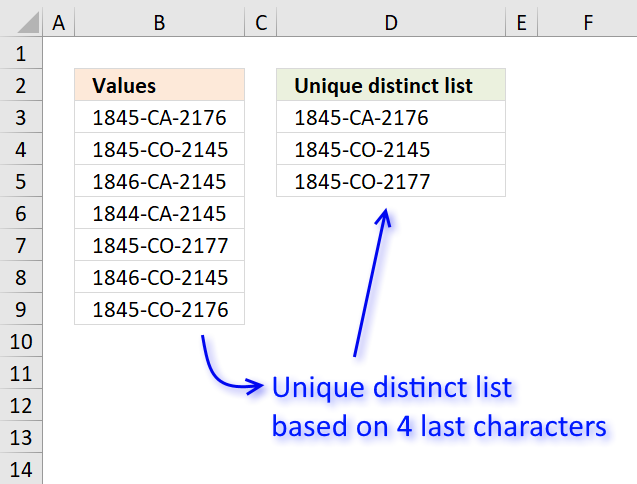The picture above shows an array formula in cell D3 that extracts a unique distinct list based on the four last characters in the cell value.

=INDEX(\$B\$3:\$B\$9, MATCH(0, MMULT(TRANSPOSE(ROW(\$A\$1:A1)^0), (RIGHT(\$D\$2:D2, 4)=TRANSPOSE(RIGHT(\$B\$3:\$B\$9, 4)))*1), 0))

You can easily replace the RIGHT function with LEFT or MID function to get a unique distinct list based on other criteria.

The issue here is that the first argument in the COUNTIF function won't accept anything else than a cell range. Not even an array of constants will work.

There are exceptions o this rule, you can use the OFFSET function in the first argument, however that won't be much help in this case.

To enter an array formula, type the formula in cell B3 then press and hold CTRL + SHIFT simultaneously, now press Enter once. Release all keys.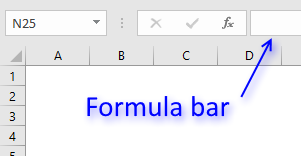The formula bar now shows the formula with a beginning and ending curly bracket telling you that you entered the formula successfully.

Don't enter the curly brackets yourself, they appear automatically.

### Explaining formula in cell D3

Use "Evaluate Formula" on tab "Formula" on the ribbon to go through the formula in small steps.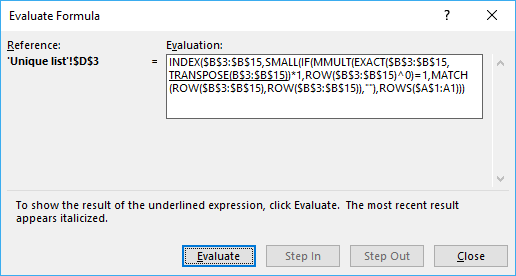#### Step 1 - Compare previous values with source list

This step compares previous values above the current cell with the source list.

This makes the formula extract only unique distinct values, the RIGHT function extracts the four last characters in each cell.

RIGHT(\$D\$2:D2, 4)=TRANSPOSE(RIGHT(\$B\$3:\$B\$9, 4))

becomes

"list"={"2176", "2145", "2145", "2145", "2177", "2145", "2176"}

returns

{FALSE, FALSE, FALSE, FALSE, FALSE, FALSE, FALSE}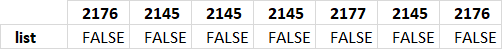Remember that in cell D3 there are no previous values except the header value.

\$D\$2:D2 is an absolute and relative cell reference. This makes it expand as the formula is copied to cells below. Make sure to copy the cell, not the formula.

#### Step 2 - Sum values column-wise

The formula needs to know which values have been displayed and which has not. It returns an array with the same size as the source list, 1 indicates that the value has been shown and 0 (zero) indicates it has not been shown.

The MMULT function is able to sum array values column-wise or row-wise depending on how you use it, you need two arguments in the MMULT function. Read more about the MMULT function here.

MMULT(TRANSPOSE(ROW(\$A\$1:A1)^0), (RIGHT(\$D\$2:D2, 4)=TRANSPOSE(RIGHT(\$B\$3:\$B\$9, 4)))*1)

becomes

MMULT(1, ({FALSE, FALSE, FALSE, FALSE, FALSE, FALSE, FALSE})*1)

becomes

MMULT(1, {0, 0, 0, 0, 0, 0, 0}))

and returns {0, 0, 0, 0, 0, 0, 0}.

This array tells the formula that all values can be extracted in cell D3, in other words no value in the source list have been displayed in cells above yet.

The calculation seems pointless in cell D3, however, it uses a cell reference that expands so the array becomes more and more advanced in cells further down.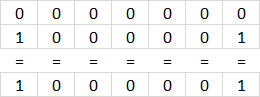For example, in cell D4 the array becomes:

MMULT({1,1},{0,0,0,0,0,0,0;1,0,0,0,0,0,1})

and returns {1,0,0,0,0,0,1}.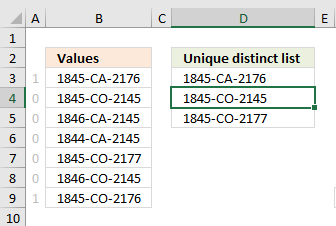The array tells the formula not to get the first and last value in cell range B3:B9 because 1845-CA-2176 has been displayed in D3.

1845-CA-2176 and 1845-CO-2176 share the same last four characters.

The picture to the right shows the array next to cell values in B3:B9.

#### Step 3 - Identify position of the first 0 (zero) in array

The MATCH function returns the relative position of a given value in an array or cell range.

MATCH(0, MMULT(TRANSPOSE(ROW(\$A\$1:A1)^0), (RIGHT(\$D\$2:D2, 4)=TRANSPOSE(RIGHT(\$B\$3:\$B\$9, 4)))*1), 0)

becomes

MATCH(0, {0,0,0,0,0,0,0}, 0)

and returns 1.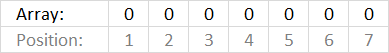Remember that this is for cell D3.

If we continue with the formula in cell D4:

MATCH(0, {1,0,0,0,0,0,1}, 0)

and returns 2. The first 0 (zero) in the array is in position 2.

#### Step 4 - Return value in given position

The formula in cell D3 is:

INDEX(\$B\$3:\$B\$9, MATCH(0, MMULT(TRANSPOSE(ROW(\$A\$1:A1)^0), (RIGHT(\$D\$2:D2, 4)=TRANSPOSE(RIGHT(\$B\$3:\$B\$9, 4)))*1), 0))

INDEX(\$B\$3:\$B\$9, 1)

and returns the value in \$B\$3 which is 1845-CA-2176 to cell D3.

If we move on to cell D4 the formula is:

INDEX(\$B\$3:\$B\$9, 2)

and returns the value in cell \$B\$4 to cell D4 which is 1845-CO-2145.